Mobile QR Code1. (Department of Electrical and Computer Eng., and Inter-University Semiconductor Research Center, Seoul National University, Seoul, Korea)

SNN, analog synapse, analog switch, neuromorphic, synaptic array

## I. INTRODUCTION

Analog synaptic devices are being investigated as one of the most important parts of neuromorphic systems because biological synapses play a role in signal transmission and memory effect (1). Recently, several types of emerging electronic synapse devices such as phase-change memory (PCRAM) (2), resistive change memory (RRAM) (3,4), ferroelectric devices (5), and FET-based devices (6,7,8) have been proposed to biological synapses. These synaptic devices, such as RRAM, MRAM, and NOR-FLASH, each have their advantages and disadvantages. The high current operation, a poor characteristic variation, reliability, and a requirement of selectors on RRAM and scalability on MRAM and NOR-FLASH are known issues. But The devices which currently used in mass production are NAND flash memory and logic transistors. We believe that we need a way to realize analog synapses with best known process and design capabilities based on those mass-production experiences. I think that it would be good to make NAND-flash with a similar structure to the standard cell of the logic CMOS device. Therefore, in this paper, we propose an analog switch type synapse using a NAND flash device and a pMOSFET.

## II. NAND FLASH MEMORY FOR SYNAPSTIC DEVICE

### 1. NAND Array for VMM

A method of constructing a synapse array using NAND cell strings has been steadily proposed due to its scalability and mass productivity. For VMM operation, all cells except the target cell are connected with the corresponding NAND cell string by applying pass voltage(V$_{\mathrm{pass}}$) as shown in Fig. 1.

Fig. 1. V$_{\mathrm{Read}}$ is applied for the output of synapse #0, otherwise V$_{\mathrm{Ppass}}$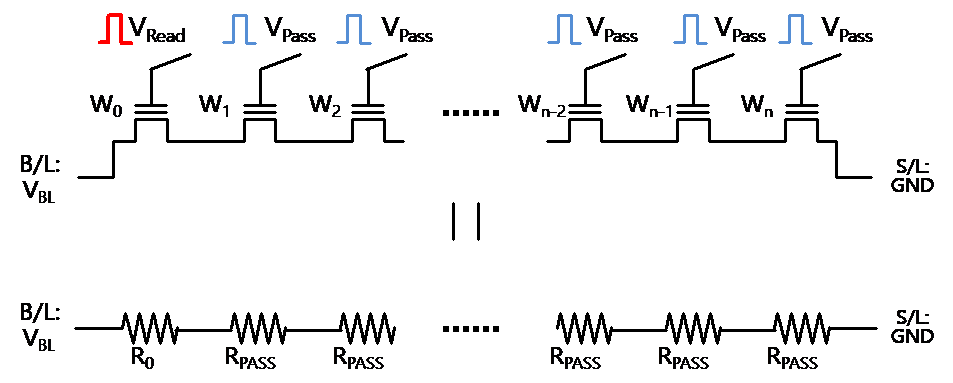For the weighted sum operation of the output neuron, a complex circuit is required to sequentially apply V$_{\mathrm{read}}$ to all cells in the string and finally calculate the weighted sum. This approach also requires an additional circuit in complex neural networks that do not know when the input signal will come as Fig. 2.

Fig. 2. V$_{\mathrm{Read}}$ is applied for the output of synapse #0, otherwise V$_{\mathrm{pass}}$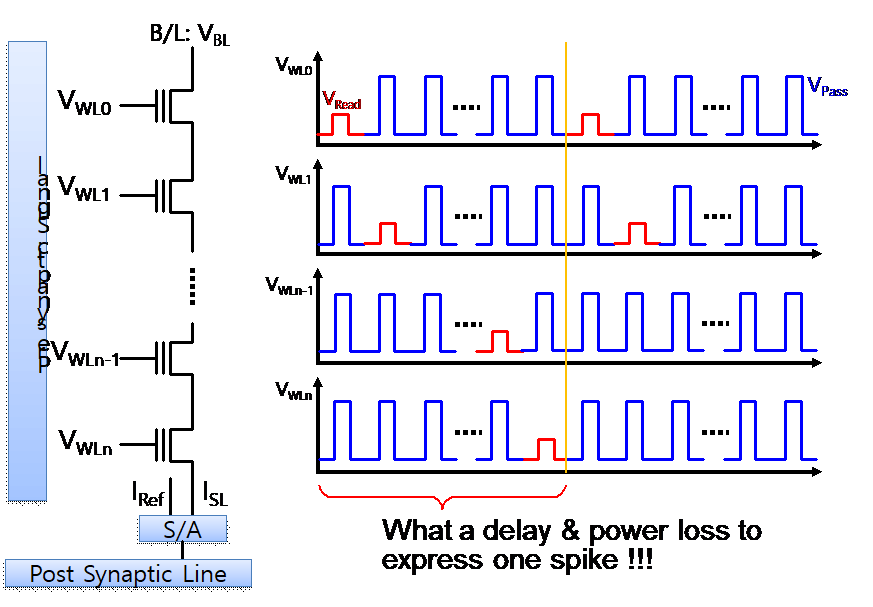The pre-synaptic signal is applied to V$_{\mathrm{read}}$ and V$_{\mathrm{pass}}$ according to the system clock and compares the amount of current flowing through the string in the sense amp. The value corresponding to the entire synapse cell needs to be accumulated through the adder before going to the postsynaptic line. This operation method is inefficient because it requires the additional signal formation circuits to generate pre-synaptic signals and compare and calculate string out currents. A different algorithm is required to use NAND flash memory as a synaptic device.

## III. ANALOG SWITCH FOR SYNAPSE

### 1. NAND String with pMOSFET for Connecting

Accumulated charge of synapse array with NAND can represent the weighted sum of neuron input in Fig. 3(a). The NAND array is broken when one cell is turned off, so it is necessary to preserve the current. If a specific route is connected by pMOSFET, a current path can be formed Fig. 3(b). If a specific resistance is used instead of pMOSFET, it can make a disturbance on NAND cell output.

We can derive the total current of this series approximately. For the derivation of a relation between synapse series current and each synapse weight. We assume binary weight system, weight=0 or 1. k$^{\mathrm{th}}$ synapse cell is on state for total n cells series otherwise off state. Equations describing total NAND string current (I$_{\mathrm{total}}$) with applied the pre-synaptic input voltage (V$_{\mathrm{GS}}$) and bit line voltage (V$_{\mathrm{BL}}$) are listed below:

##### (1)
$$I_{D S}=\mu_{n} \frac{\varepsilon_{o x}}{t_{o x}} \frac{W}{L}\left(V_{G S}-V_{T H}\right) V_{D S} \text { for } V_{G S} \gg V_{D S}$$

##### (2)
$$I_{\text {total}}=\frac{V_{B L}}{2 R_{o n} n-k}=V_{B L}\left(2 R_{o n} n-k\right)^{-1}$$

##### (3)
$$\begin{array}{l} I_{\text {total}} \cong \frac{V_{B L}}{R_{o f f} n}+\frac{V_{B L}}{4 R_{o n}^{2} n^{2}} k \\ \text { at } R_{o n}=\frac{1}{\mu_{n}} \frac{t_{o x}}{\varepsilon_{o x}} \frac{L}{W} V_{o v} \end{array}$$

Where R$_{\mathrm{off}}$ denotes off-state resistance value for no pre-synaptic input signal case, R$_{\mathrm{on}}$ is for having a pre-synaptic input signal (V$_{\mathrm{ov}}$). I$_{\mathrm{DS}}$ is current for each synapse cell and Vov is the difference between V$_{\mathrm{GS}}$ and threshold voltage(V$_{\mathrm{th}}$). We can define total resistance R$_{\mathrm{total }}$and total current I$_{\mathrm{total}}$.

There is interference due to each cell V$_{\mathrm{DS}}$ differences which can occur depending on the position of each cell in the array. But we can assume V$_{\mathrm{DS}}$ is the same at each synapse cell. It because V$_{\mathrm{DS}}$ is very small value due to bit line voltage(V$_{\mathrm{BL}}$) dividing by number of synapse cells. And R$_{\mathrm{off}}$ value is not big different from R$_{\mathrm{on}}$ also.

Eq. (3) is Taylor approximation to 1$^{\mathrm{st}}$ order. We assume total number of synapse cells is big enough to the number of on-state cells (n{\textgreater}{\textgreater}k).

### 2. Characteristics of Synapse

Fig. 4(a) is schematic of a proposed synapse cell. The gates of NAND and pMOSFET are connected to the same input. When current flows through the NAND cell, there is no current distortion because the pMOSFET is off. At the same time, if the synapse input is 0, the pMOSFET will operate to provide the current. Fig. 4(b) is the layout of the proposed synapse. This is similar to the form of a CMOS logic circuit. The similarity of the layout is a big advantage in the fabrication process in terms of process variation when the logic cell and this synapse cell process at the same time. The input value is represented by the number of spikes. In addition, V$_{th}$ change according to the amount of charge trap of NAND cell can represent the weight in Fig. 4(c).

Fig. 4. single synapse cell structure (a) Schematic of 1 synapse cell with 1 floating gate (b) Design layout of 1 synapse cell (c) synapse cell characteristic by V$_{th}$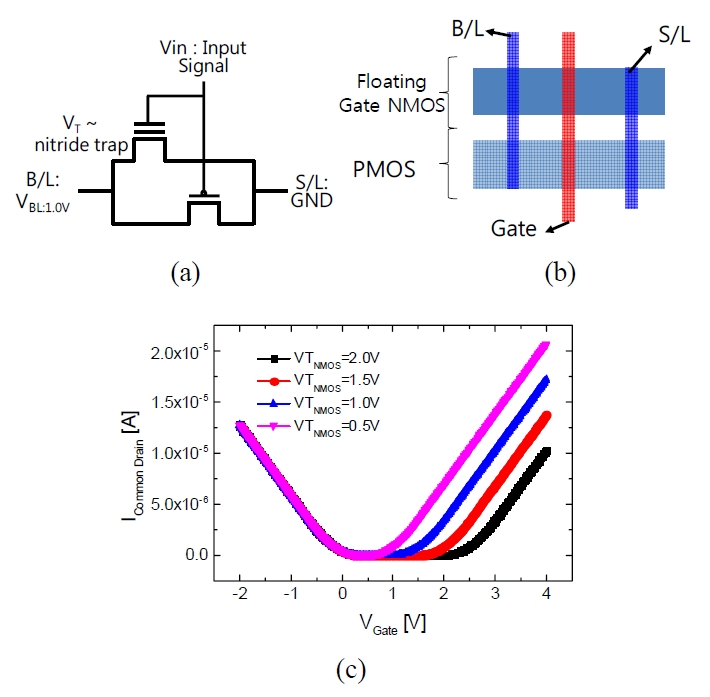### 3. Scaling Comparison

Generally, NAND and NOR cell sizes are 4F$^{2}$ and 10F$^{2}$. However, the proposed synapse device inevitably requires twice the size of the NAND due to the presence of a pass transistor. In addition, since pMOSFET and nMOSFET are connected, additional area loss due to well boundary impact is expected. In the end, an area of about 8F2 + ${\alpha}$ is expected, and it is similar in terms of NOR structure and size.

## IV. CIRCUIT OPERATION ANALYSIS

In the circuit operation analysis for VMM: Using the 10 cells synapse devices, we perform the spice simulation of VMM operation. As in Fig. 5(a), excitatory synapse and inhibitory synapse is made using 20 synapses with current mirrors, and the voltage of membrane capacitance of Integrate-and-Fire (I&F) system is measured.

To do this, we randomly generate a weight value between -1 and 1 and an input signal between 0 and 100. The input pulse uses the rate coding method and V$_{th}$ is adjusted to the second decimal point. Then weighted sum value calculation is listed below:

##### (4)
$$\text{Weighted Sum} \\ =\sum \frac{\text{Random Input}(0 \sim 100)}{100} \times \text{Random weight} (-1 \sim 1)$$

And V$_{th}$ value is listed for the inference below:

##### (5)
\begin{aligned} &\text{For positive weight value :}\\ &V_{th}=\text{(2.0-random weight) on excitatory synapse} \\ &V_{th}=\text{2.0 on inhibitory synapse}\\ &\text{For negative weight value :}\\ &V_{th}=\text{2.0 on excitatory synapse}\\ &V_{th}=\text{(2.0+random weight) on inhibitory synapse} \end{aligned}

As a result of Fig. 5(b), the weighted sum and membrane potential has a 99% correlation value. For the perfect definition of synapse weight, membrane voltage have to be exact linear relation. But there is some variation. This comes from assumptions in Eqs. (1)-(3). VDS cannot be exact same at each synapse node. And Itotal is not perfect linear function even in region n>>k. These two assumption can lead an accuracy degradation. Even though existing variation, membrane voltage and weighted sum value has a good correlation.

Fig. 5. VMM SPICE simulation setup and result (a) 10 excitatory and 10 inhibitory synapses are implemented with current mirrors and membrane capacitor for neuron input, (b) R$^{2}$ value is near 0.99 for a randomly generated weighted sum.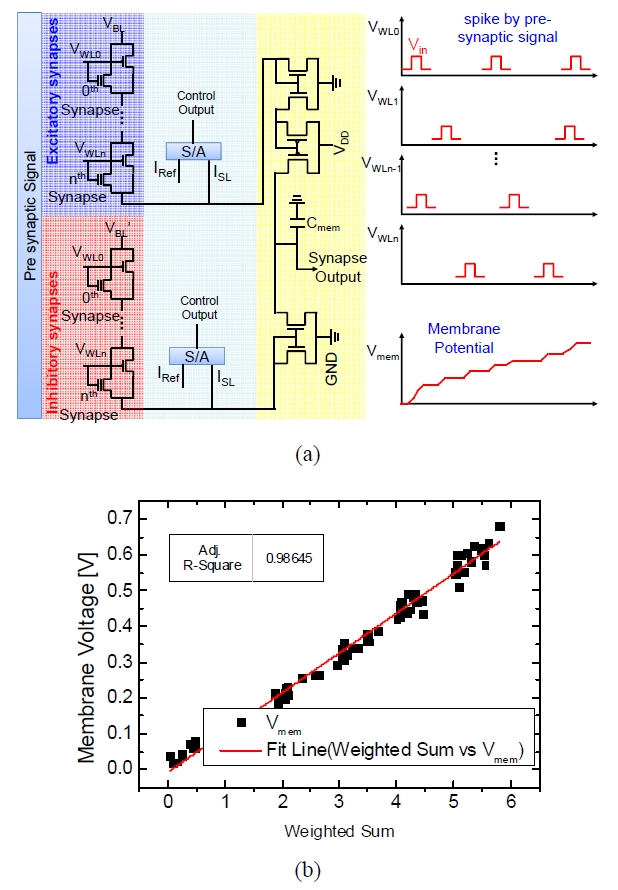The precision of the NAND cell has a big impact on VMM accuracy. To check the VMM accuracy, we perform the simulation by the number of V$_{th}$ offering of NAND cell. The R$^{2}$ value is 95% with 3bit NAND cell. There is a significant accuracy degradation for under 2 bits NAND cell in Fig. 6. In order to secure accuracy, it is necessary to make a sufficient V$_{th}$ range.

Fig. 6. (a) VMM correlation chart between membrane potential which is output of synapse and weighted sum value by # of weights on NAND, (b) VMM correlation value by # of weights on NAND.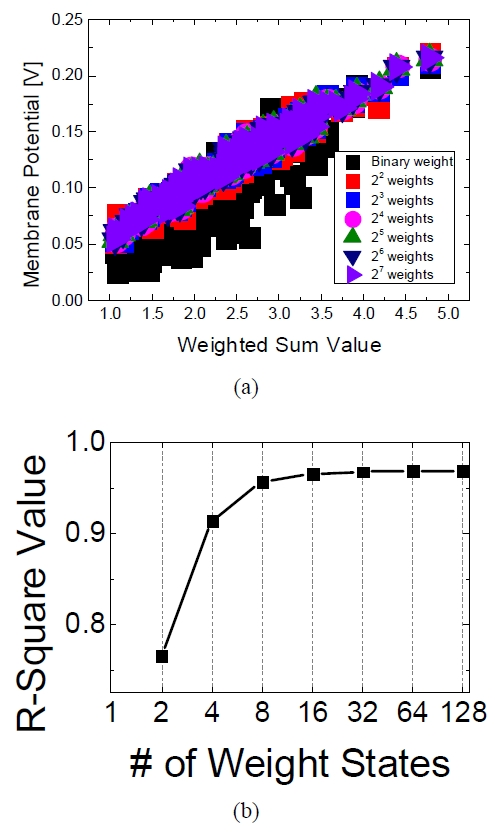## V. BENCH MARKING RESULT WITH MNIST

Based on this synapse array, we examine the difference in recognition rate according to ANN's accuracy and cell variation of the proposed synapse. For this purpose, 8×8 hand-written digit data are used as shown in Table 1 (9).

Table 1. 8×8 hand-written digit data from Scikit-Learn (9)

 Classes 10 Samples per class ~180 Total Samples 1,797 Dimensionality 64 (8×8) Features Integers 0-16

Fig. 7 is the entire VMM architecture for MNIST benchmarking. Program and erase operation for weight projection is the same as usual NAND memory architecture. The Only capacitor is added for current integration. This membrane capacitor will connect to the neuron circuit to fire postsynaptic spike.

First, 1-layer fully-connected-network, which is a reference, performs learning using SciKit-Learn sample code (10). The recognition rate was 86%. The obtained weight value infers to each NAND cell by value of threshold voltage based on Eq. (3). And the 1,797 rate coded input data is converted into an input signal by modulated input frequency. SPICE simulation is performed by HSPICE simulator. After performing spice simulation, we pick up the largest voltage value of the membrane capacitor. It is the result of model recognition. We complete the correlation plot as shown in Fig. 8(a) and (b). The recognition rate has a difference by 3% compared with ANN's.

Fig. 8. Correlation plot of 8×8 MNIST by spice simulation (a) result of 1-layer FCN by ANN, (b) result of 1-layer FCN by SPICE simulation with weight projection which is from ANN result.## VI. CONCLUSIONS

The analog switch type synapse using the proposed NAND array and pMOSFET has the advantage that the existing NAND program / erase mechanism can be used without any change in the mass production process. At the same time, it has the advantage of using same architecture of standard cell unit on an industry process. At the same time having a chance of smaller area than silicon-based synapse due to processing with logic and NAND synapse unit. And SPICE simulation of this synapse inference verification of FCN network resulted in 83% recognition rate, which is 3% lower than reference value of ANN.

### ACKNOWLEDGMENTS

This work was supported by the Brain Korea21 Plus project in 2019 and Nano – Material Technology Development Program through the National Research Foundation of Korea funded by the Ministry of Science, ICT and Future Planning (2016M3A7B4910348).

### REFERENCES

1
Kandel E. R., 2001, The molecular biology of memory storage: a dialogue between genes and synapses, Science, Vol. 294, No. 5544, pp. 1030-10382
Suri M., 2011, Phase change memory as synapse for ultra-dense neuromorphic systems: Application to complex visual pattern extraction, 2011 International Electron Devices Meeting, Washington, DC, pp. 4.4.1.-4.4.4.3
Jo Sung Hyun, Chang Ting, Ebong Idongesit, Bhadviya Bhavitavya B., Mazumder Pinaki, Lu Wei, 2011, Nanoscale memristor device as synapse in neuromorphic systems., Nano Lett4
Kwon Min-Woo, Baek Myung-Hyun, Park Jungjin, Kim Hyungjin, Hwang Sungmin, Park Byung-Gook, 2017, CMOS Analog Integrate-and-fire Neuron Circuit for Driving Memristor based on RRAM, Journal of Semiconductor Technology and Science, Vol. 17, No. 2, pp. 174-1795
Chanthbouala A., Garcia V., Cherifi Ryan, Bouzehouane K., Fusil S., Moya X., Xavier S., Yamada Hiroyuki, Deranlot Cyrile, Mathur Neil D., Bibes Manuel, Barthelemy A., Grollier Julie, Oct 2012, A ferroelectric memristor, Nature Materials, Vol. 11, No. 10, pp. 860-8646
Kim H., Park J., Kwon M., Lee J., Park B., March 2016, Silicon-Based Floating-Body Synaptic Transistor With Frequency-Dependent Short- and Long-Term Memories, in IEEE Electron Device Letters, Vol. 37, No. 3, pp. 249-2527
Lin Y., 2018, A Novel Voltage-Accumulation Vector-Matrix Multiplication Architecture Using Resistor-shunted Floating Gate Flash Memory Device for Low-power and High-density Neural Network Applications, 2018 IEEE International Electron Devices Meeting (IEDM), San Francisco, CA, pp. 2.4.1-2.4.4.8
Kim Sangwook, Kim Sangwan, 2018, High-performance Recessed-channel Reconfigurable Field-effect Transistor using Si-Ge Heterojunction, Journal of Semiconductor Technology and Science, Vol. 18, No. 3, pp. 392-3959
Dua D., Graff C., UCI Machine Learning Repository [http://archive.ics.uci.edu/ml]10
Pedregosa F., 2011, Scikit-learn: Machine Learning in Python, JMLR## Author

Su-Hyeon Kim received the B.S., M.S. degrees in the Department of Physics from Yonsei University, Seoul, Korea, in 2005, and 2008, respectively.

In 2008, he joined at Samsung Electronics, where he has been working in the area of logic technology development of Semiconductor R&D Center.

He is currently working toward the Ph.D. degree in electrical and computer engineering, Seoul National University, Seoul, Korea.

His interests include a synaptic device for neuromorphic systems.

Myung-Hyun Baek received the B.S. degree in Electrical Engineering in 2013 from Seoul National University (SNU), Seoul, Korea.

He is currently working toward the Ph.D. degree in the department of electrical and computer engineering, Seoul National University, Seoul, Korea.

His main research interests are nonvolatile memory technologies and neuromorphic systems.

Sungmin Hwang received the B.S., M.S. degree in Hanyang University in 2014.

He is currently working toward Ph.D. degree in the department of electrical and computer engineering, Seoul National University, Seoul, Korea.

His research interests include nanoscale silicon devices, and neuromorphic systems.

Taejin Jang received the B.S., degree in Electrical Engineering in 2016 from Seoul National University (SNU), Seoul, Korea.

Kyung Jang received the B.S., degree in in 2014 from SungKyun- Kwan University (SKKU), Suwon, Korea.

He is currently working toward Ph.D. degree in electrical engineering in Seoul National University, Seoul, Korea.

Byung-Gook Park received his B.S. and M.S. degrees in electronics engineering from Seoul National University (SNU) in 1982 and 1984, respectively, and his Ph.D. degree in electrical engineering from Stanford University in 1990.

From 1990 to1993, he worked at the AT&T Bell Laboratories, where he contributed to the development of 0.1 micron CMOS and its characterization.

From 1993 to 1994, he was with Texas Instruments, developing 0.25 micron CMOS.

In 1994, he joined SNU as an assistant professor in the School of Electrical Engineering (SoEE), where he is currently a professor.

In 2002, he worked at Stanford University as a visiting professor, on his sabbatical leave from SNU.

He led the Inter-University Semiconductor Research Center (ISRC) at SNU as the director from 2008 to 2010.

His current research interests include the design and fabrication of nanoscale CMOS, flash memories, silicon quantum devices and organic thin film transistors. He has authored and co-authored over 1000 research papers in journals and conferences.

Prof. Park has served as a committee member on several international conferences including Micro processes and Nanotechnology, IEEE International Electron Devices Meeting, International Conference on Solid State Devices and Materials, and IEEE Silicon Nanoelectronics Workshop and served as an Editor of IEEE Electron Device Letters.

He received “Best Teacher” Award from SoEE in 1997, Doyeon Award for Creative Research from ISRC in 2003, Haedong Paper Award from the Institute of Electronic Engineers of Korea (IEEK) in 2015.

He has served as a Committee Member on several international conferences, including Microprocesses and Nanotechnology, IEEE International Electron Devices Meeting, International Conference on Solid State Devices and Materials, and IEEE Silicon Nanoelectronics Workshop (the Technical Program Chair in 2005, the General Chair in 2007).

He is currently serving as a Cooperative Vice President of IEEK and the Board Member of the IEEE Seoul Section.